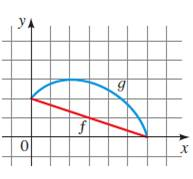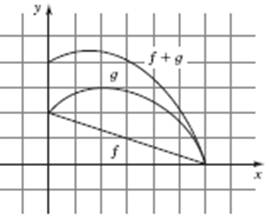# the graph of f + g### Precalculus: Mathematics for Calcu...

6th Edition
Stewart + 5 others
Publisher: Cengage Learning
ISBN: 9780840068071### Precalculus: Mathematics for Calcu...

6th Edition
Stewart + 5 others
Publisher: Cengage Learning
ISBN: 9780840068071

#### Solutions

Chapter 2.6, Problem 15E
To determine

## To sketch : the graph of f+g

Expert Solution

### Explanation of Solution

Given information : The graph is provided belowGraph : To sketch the graph of f+g

Use pencil to draw the f+g graph for provided graphInterpretation : The above graph shows the f+g graph

The

### Have a homework question?

Subscribe to bartleby learn! Ask subject matter experts 30 homework questions each month. Plus, you’ll have access to millions of step-by-step textbook answers!